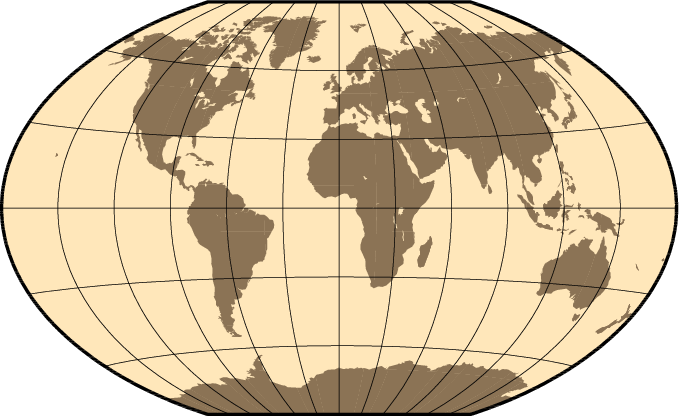# Reprojecting maps

Dear community,
does anyone have a recipe/example of how to reproject a map, e.g. from lat/lon to Robinson (for my use case just global is enough)? Please note I do not just want to plot (cf. GeoMakie), but get a Raster or matrix back.

Essentially in the MWE below I would like to avoid `using RCall`:

``````using GLMakie
using RCall
using Rasters
@rlibrary terra

function countryRaster(; res=0.5)
shapefile_name = "country_borders.shp"
countries = Shapefile.Handle(shapefile_name).shapes
world = Rasters.rasterize(countries; to = Extents.Extent(X = (-180, 180), Y = (-90, 90)), res=res, missingval=0, fill=1, shape=:line)
return world.data.data
end

function proj(grid, outcrs="+proj=robin +datum=WGS84")# +units=m +no_defs")
#grid = permutedims(grid, (2,1))
jras = R"""
terra::rast(\$(permutedims(grid, (2,1))), crs = "+proj=longlat +datum=WGS84 +no_defs", extent=ext(rast())) %>% terra::project(\$outcrs) %>% as.matrix(wide=T)
""" |> rcopy
return permutedims(jras, (2,1))
end

heatmap(countryRaster() |> proj)

``````

Thanks!

See GMT.jl

Thanks! Could you provide an example? From the docs I see mainly the plotting. Thanks in advance!

`Rasters.reproject` is the function in Rasters.jl, apologies I dont have time to write an example right now.

But have you tried that? The docs should specify the arguments you need.

Thanks, no had only tried Rasters.resample, but I could not get it to work.

Rasters.reproject gives this

``````julia> Rasters.reproject(ProjString("+proj=robin +datum=WGS84"), ras)
ERROR: StackOverflowError:
Stacktrace:
 reproject(source::Nothing, target::ProjString, dim::X{Colon}, vals::StepRangeLen{Float64, Base.TwicePrecision{Float64}, Base.TwicePrecision{Float64}, Int64}) (repeats 79984 times)
``````

where `ras` was the return of `world` from the `countryRaster` function above.

Any idea?
Thanks,
Markus

Arrg sorry i was away from a computer `resample` is what you want:

``````res = 0.5
shapefile_name = "country_borders.shp"
countries = Shapefile.Handle(shapefile_name).shapes
world = Rasters.rasterize(countries; to = Extents.Extent(X = (-180, 180), Y = (-90, 90)), res, missingval=0, fill=1, shape=:line)
crs = ProjString("+proj=robin +datum=WGS84")
wsg84_world = resample(world, res; crs)
Makie.heatmap(wsg84_world)
``````

I do want to make the syntax a little easier to understand for resample.

In future it will be better to use ArchGDAL.jl or Proj.jl to reproject the shapefile instead of resampling the raster, but my 2 PRs to make both of those things work on any geometries (like Shapefile.jl objects) are not quite finished.

Thanks, yes in the real example I have a raster in the first place. Just used the county borders as a transparent MWE.

Unfortunately, I cannot reproduce, but get the error (Trace cut at ). Also I note that your output does not seem to be reprojected to Robinson.

``````julia> wsg84_world = Rasters.resample(world, reso; crs)
ERROR: BoundsError: attempt to access 0-element Vector{String} at index 
Stacktrace:
 getindex
@ .\array.jl:924 [inlined]
 first
@ .\abstractarray.jl:404 [inlined]
@ Rasters C:\Users\mreichstein\.julia\packages\Rasters\wUuLU\src\sources\gdal.jl:231
 dims(raster::ArchGDAL.RasterDataset{Int64, ArchGDAL.Dataset}, crs::Nothing, mappedcrs::Nothing)
@ Rasters C:\Users\mreichstein\.julia\packages\Rasters\wUuLU\src\sources\gdal.jl:157
 dims
@ C:\Users\mreichstein\.julia\packages\Rasters\wUuLU\src\sources\gdal.jl:142 [inlined]
 copyto!(dest::ArchGDAL.RasterDataset{Int64, ArchGDAL.Dataset}, bc::Base.Broadcast.Broadcasted{DimensionalData.DimensionalStyle{Base.Broadcast.DefaultArrayStyle{2}}, Tuple{Base.OneTo{Int64}, Base.OneTo{Int64}, Base.OneTo{Int64}}, typeof(identity), Tuple{Raster{Int64, 2, Tuple{X{Projected{Float64, StepRangeLen{Float64, Base.TwicePrecision{Float64}, Base.TwicePrecision{Float64}, Int64}, DimensionalData.Dimensions.LookupArrays.ForwardOrdered, DimensionalData.Dimensions.LookupArrays.Regular{Float64},
DimensionalData.Dimensions.LookupArrays.Intervals{DimensionalData.Dimensions.LookupArrays.Start}, DimensionalData.Dimensions.LookupArrays.NoMetadata, Nothing, Nothing, X{Colon}}}, Y{Projected{Float64, StepRangeLen{Float64, Base.TwicePrecision{Float64}, Base.TwicePrecision{Float64}, Int64}, DimensionalData.Dimensions.LookupArrays.ForwardOrdered, DimensionalData.Dimensions.LookupArrays.Regular{Float64}, DimensionalData.Dimensions.LookupArrays.Intervals{DimensionalData.Dimensions.LookupArrays.Start}, DimensionalData.Dimensions.LookupArrays.NoMetadata, Nothing, Nothing, Y{Colon}}}}, Tuple{}, Matrix{Int64}, Nothing, DimensionalData.Dimensions.LookupArrays.NoMetadata, Int64}}})
``````

Sorry I’m not at the computer. But maybe the example in the front page of the GMT.jl site

``````coast(region=:global, proj=:Winkel, frame=:g, area=10000,
land=:burlywood4, water=:wheat1, show=true)
``````Yeah, it looks like there may be a bug in `resample` somewhere, but we may also be on different versions of some other package for you to get that error.

Can you make a Github issue? That’s usually the best thing to do when you get an error with Rasters.jl.

I’ll be away for a few days but having the issue there means I will get to it at some point after that.